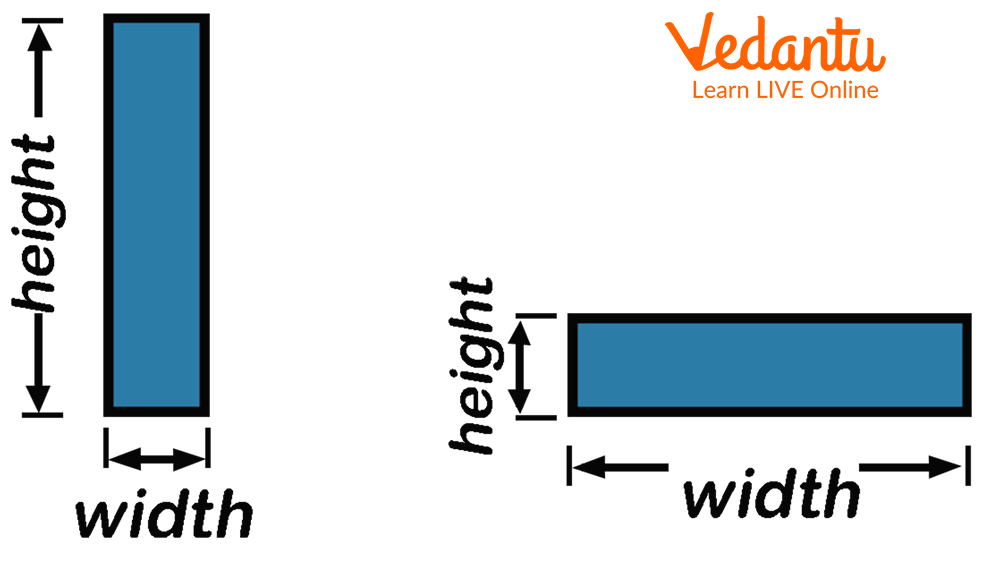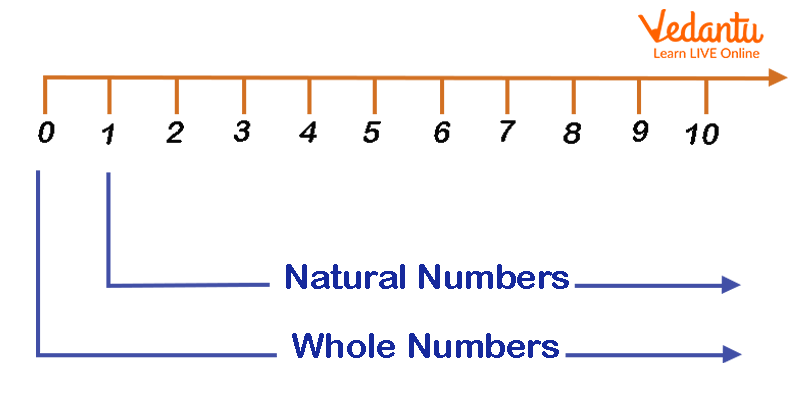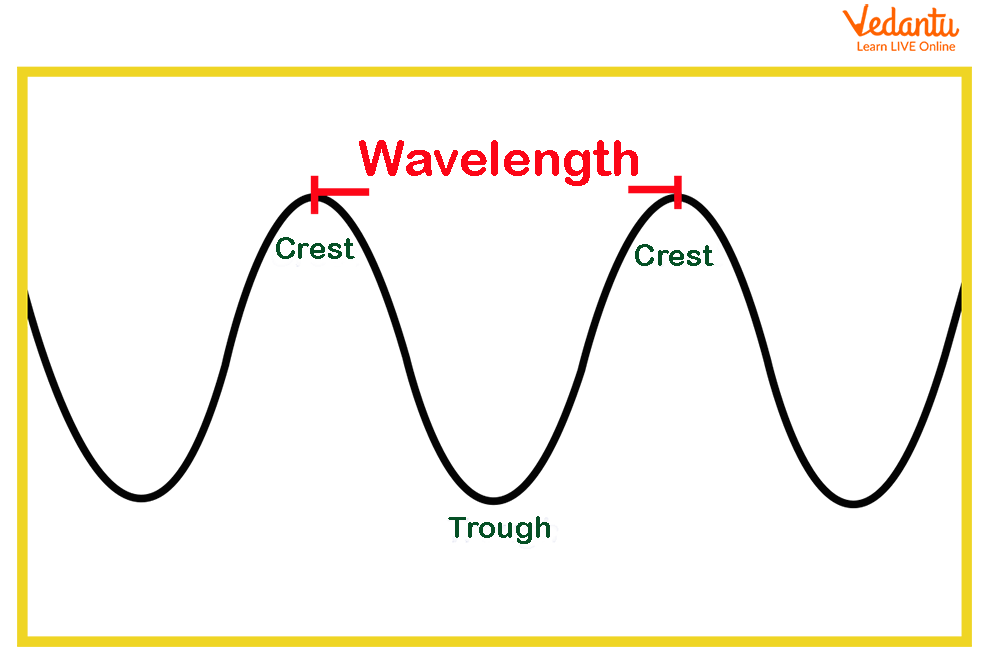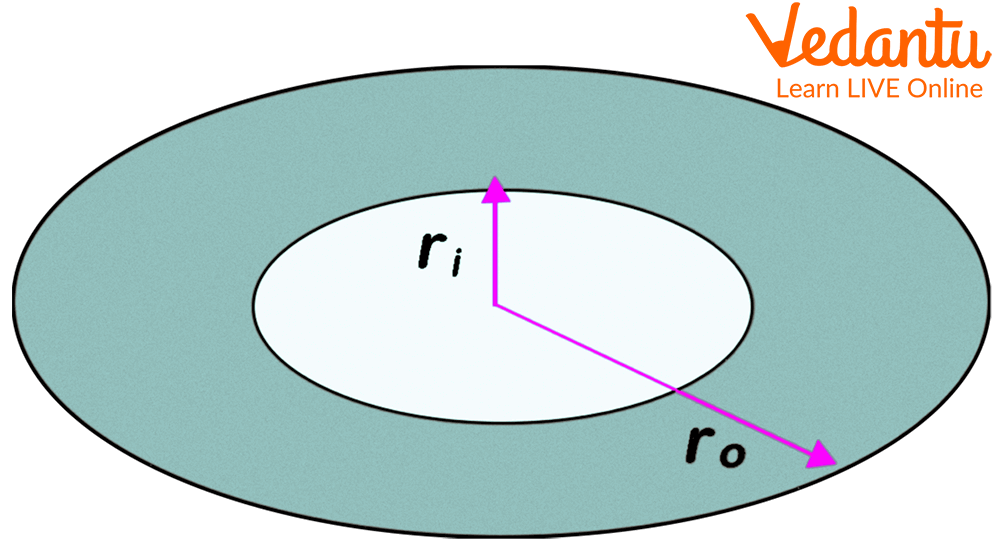Courses
Courses for Kids
Free study material
Free LIVE classes
More## Introduction to Maths Words

Mathematics is a fascinating subject, almost all natural phenomena have a Mathematical basis. Although it is a wide subject that encompasses several specialisations, kids at an early age are taught simple Mathematical concepts like subtraction, multiplication, division, and addition. But as the kids grow up, the concepts of Maths taught widen, the simple Mathematical operations are then used to calculate measurements, equations, and other problems.

With all such advances, one of the common traits that are repeated is the use of words and their abbreviations in problems. The introduction of Maths words can be challenging to understand for kids. The article focuses on the description of such Maths words that start with ‘w’. The knowledge of these words can help kids develop a precise understanding of the complex Mathematical concepts.

There are several abbreviations that are used in Mathematics. Here, we have provided some of the words used by kids in Maths along with their definitions.

• Width

• Weight

• Whole numbers

• Winding number

• Wavelength

• Weighted average

• Washer

• Washer method

## Width

Width is abbreviated as w. Width is a parameter used to measure the distance between two edges of an object. A common concept that is taught to kids is to calculate the width of a triangle or rectangle. The image mentioned below provides a better insight of what is width.illustrating the width of the rectangle

## Weight

Weight is the measure of how heavy a body is. A more scientific definition of the term is the measure of the gravitational force acting on the body. Weight is measured in units of kilograms, grams, pounds, and milligrams.

## Whole Numbers

A whole number is defined as the set of numbers that includes all the natural numbers as well as zero. They are part of real numbers but do not include fractions, decimals, and negative numbers that are not included in real numbers. The image illustrated below shows the whole numbers.illustrating whole numbers on the number line

## Winding Number

The winding number is also known as the winding index. It is defined as the number of counterclockwise passes a closed curve in the plane makes around a given point.

## Wavelength

Wavelength is a complicated concept that is primarily used in Physics. It is defined as the distance between the two sinusoidal waves. In simpler terms, the wavelength is used to measure the distance between two waves.illustrating the wavelength of waves

## Weighted Average

It is a method for calculating the arithmetic mean of a series of data where some of the values in the set are often more important than others. A weighted average is a calculation that considers the relative value of the integers in a data collection. Each value in the data set is multiplied by a predefined weight before the final computation is completed when calculating a weighted average.

## Washer

The washer is defined as the area in between two concentric circles whose radii are different. The washer method is used to calculate the area of such circles.illustrating the concentric circles with different radii

## Conclusion

We have learnt different Maths words that start with ‘w’. Besides the keywords mentioned in the article, there are numerous other terms in Mathematics that begin with the letter ‘w’. Learning these keywords can help students widen their Maths vocabulary, especially those that begin with the letter ‘w’. As a result, a Maths dictionary is essential for all scholars. It is critical to use the dictionary for all 26 alphabets, especially on the topic of Mathematics.

Last updated date: 23rd Sep 2023
Total views: 134.7k
Views today: 3.34k

## FAQs on Glossary - Maths Words that Start with W for Kids

1. Name and define a common Mathematical term with the letter ‘a’.

A common term that is used in Mathematics is the ‘area’. The area can be defined as the space between the two-dimensional figures. Another term that is similar to the area is surface area. Surface area is defined as the sum total area of every side and face of a three-dimensional figure.

2. How can a Mathematics glossary help students?

As the concepts of Mathematics get broader, several new terms and phrases are introduced to the students. Learning these common terms and their phrases can provide students with a chance of precise understanding, apart from this, it can also help students with the ease of solving Mathematical problems. Apart from (w), there are terms with x and y that are frequently used in Maths.